﻿ 一种空间仿生柔性机器人设计与智能规划仿真方法
 机器人2022, Vol. 44Issue (3): 361-3670

LIU Wuji, JING Zhongliang, CHEN Wujun, PAN Han. Design and Intelligent Planning Simulation Method of a Space Bionic Soft Robot[J]. ROBOT, 2022, 44(3): 361-367.1. 上海交通大学航空航天学院，上海 200240;
2. 上海交通大学船舶海洋与建筑工程学院，上海 200240

Design and Intelligent Planning Simulation Method of a Space Bionic Soft Robot
LIU Wuji1, JING Zhongliang1 , CHEN Wujun2 , PAN Han1
1. School of Aeronautics and Astronautics, Shanghai Jiao Tong University, Shanghai 200240, China;
2. School of Naval Architecture, Ocean & Civil Engineering, Shanghai Jiao Tong University, Shanghai 200240, China
Abstract: Conventional space rigid-body robots are subject to defects such as limited degree of freedom and low environmental adaptability. For those problems, a soft robot based on the organism structure is designed inspired by inchworm and snake, which is applicable to space in-orbit service. Firstly, the prototype of the soft robot is built and the drive characteristics of the Ni-Ti shape memory alloy (SMA) as the actuators are studied. A visual control interface is designed and the manoeuvrability of the soft robot prototype is verified by physical experiments. Besides, the Q-learning algorithm and the corresponding reward function based on the designed soft robot structure are designed. A simulation model of the soft robot is built, and autonomous learning and planning experiment of the robot arm model based on Q-learning is completed in the simulation environment. The experiment results show that the robot arm can converge to a stable state in a short time and complete planning tasks independently. Therefore, the proposed algorithm is effective and feasible, and the reinforcement learning method has a good application prospect in the intelligent planning and control of soft robots.
Keywords: bionic robot    shape memory alloy (SMA)    Q-learning    intelligent planning

1 引言（Introduction）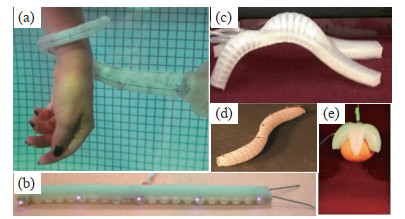图 1 仿生柔性机器人实例 Fig.1 Examples of bionic soft robots

1) 提出了一种具有高形变和耦合弯曲能力的新型柔性机器人结构，设计建造了一种仿生柔性GS-Bot机器人原型样机。利用镍钛SMA作为驱动器，使得GS-Bot机器人具有相对较高的形变和驱动力。同时通过实物实验验证了其具有可操控能力。

2) 设计了一种针对GS-Bot机器人的Q学习方法，并在仿真环境中验证了GS-Bot机器人仿真模型在训练后能够自主智能地完成目标任务。

2 GS-Bot柔性机器人系统组成（Composi- tion of the GS-Bot soft robot system）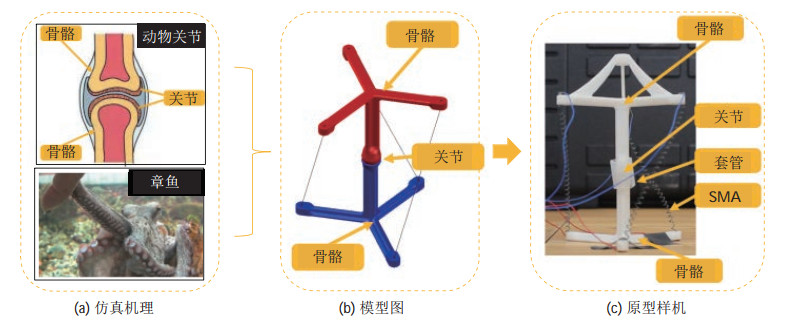图 2 机器人仿生机理和原型样机 Fig.2 Bionic mechanism and prototype of GS-Bot

GS-Bot原型样机如图 2(c)所示，每节关节由2个光敏树脂材料制成的骨架连接构成，3根SMA弹簧连接在相邻2节之间用于提供驱动力。同时，为了达到骨关节扭转效果，骨架连接处被设计为“球窝”结构，并使用橡胶管来约束偏转角的范围。GS-Bot机器人能够在传统刚体机器人扭转操作受限的非结构化空间和限制空间中发挥重要的作用。

2.1 GS-Bot系统架构组成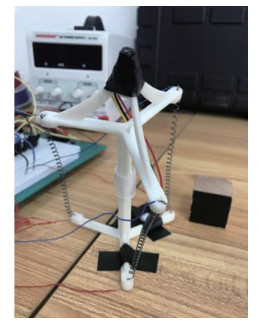图 3 机器人样机 Fig.3 GS-Bot prototype

SMA弹簧相对其他柔性材料具有相对较高的变形性能、高功重比和可恢复性，常被选为驱动器。SMA弹簧加热时会收缩，冷却后可由外力拉伸恢复到初始形状。为了测试SMA物理性能和材料的可重复性，本文使用立式和卧式橡胶拉伸试验机验证SMA弹簧受温度影响的伸缩特性及在驱动电压和不同温度方面的恢复特性。当驱动电压为3 V时，SMA弹簧对不同室温的敏感程度不大，对于不同驱动电流大小表现十分敏感，20 s以后才开始产生较大形变。当驱动电压为6 V时，SMA从第10 s开始急剧变化，直到第20 s趋于稳定。以上结果表明SMA的形变性质对于电压变化更加敏感。

3 GS-Bot机器人智能规划仿真（Simulation of GS-Bot intelligent planning）

GS-Bot机器人的姿态与运动规划控制主要通过SMA弹簧的伸缩带动骨节间的角度偏转来实现，实际运动具有非常大的非线性。因此将GS-Bot机器人简化为2维平面中的机器臂连杆模型进行自主规划仿真，如图 4所示。利用机器臂的关节角度控制机器臂的位置和姿态。图 4中红色部分代表 2根机器臂连杆，通过连杆间的角度变化控制机器臂姿态和端点位置。2维平面内设置一个蓝色目标物，用于表示机器臂被期望到达的目标位置。仿真模型中$O(x_{0}, y_{0})$表示机器臂基座固定点，$l_{1} $$l_{2} 分别表示2根机器臂连杆的长度。连杆端点分别为基座固定点 O(x_{0}, y_{0} ) 、第1节连杆端点 E_{1} (x_{1}, y_{1}) 和第2节连杆的末端点 E_{2} (x_{2}, y_{2}) 。2根连杆可以分别围绕点 O$$ E_{1}$在2维平面中以任意角度旋转，直到机器臂末端点$E_{2}$自主到达蓝色目标物区域$G(x_{G}, y_{G})$。其中，\alpha \beta 分别表示2根连杆与水平轴的夹角，用于表示2根连杆的旋转角度。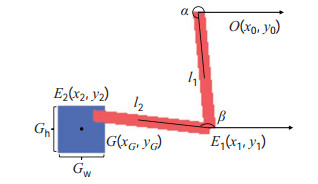图 4 简化仿真模型示意图 Fig.4 The schematic diagram of GS-Bot simplified simulation model 为描述仿真环境中机器臂的姿态和位置，本文选用2维物理量进行定量分析：机器臂长度和旋转角度。因此可得到3个端点的点坐标关系式如下：  \begin{equation} \begin{aligned} (x_{1}, y_{1}) &=(l_{1} \cos \alpha, l_{1} \sin \alpha) +(x_{0}, y_{0}) \\[-3pt] (x_{2}, y_{2}) &=(l_{2} \cos \beta, l_{2} \sin \beta) +(x_{1}, y_{1}) \end{aligned} \end{equation} (1) 通过3个端点 O E_{1} $$E_{2} 能够准确且完整地描述机器臂的位置和姿态信息。 本文采用Q学习智能规划方法对机器臂进行自主学习和驱动，通过所建立的奖励函数对仿真智能体进行训练，并最终达到机器臂自主规划和驱动的目的。在Q学习中，针对被控对象智能体，定义在 t 时刻智能体采取的动作为 a_{t} ，对应状态和奖励分别为 s_{t}$$ r_{t}，衰减因子为$\gamma \in (0, 1)$。同时定义智能体的策略函数为$\pi ( {a_{t}} |s_{t} )$，表示智能体在状态$s_{t}$下选取动作$a_{t}$的概率。算法如图 5所示。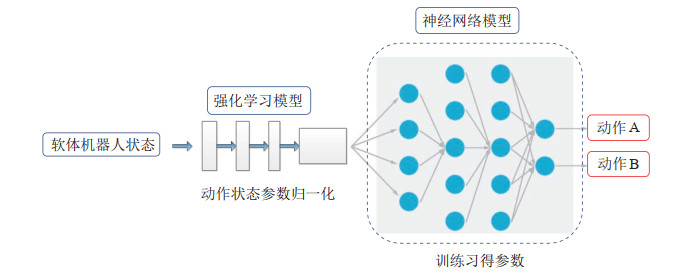图 5 基于Q学习的规划算法 Fig.5 Planning algorithm based on Q-learning

 $\begin{equation} P_{{\rm r}} = \begin{cases} \pi ( {a_{t}} |s_{t}) <1 \\ \sum \pi ( {a_{t}} |s_{t}) =1 \\ \end{cases} \end{equation}$ (2)

 $\begin{equation} P_{{\rm d}} =\pi ( {a_{t}} |s_{t}): s\to a \end{equation}$ (3)

 $\begin{equation} V_{\pi} (s)=E_{s_{t}, s_{t+1}, \cdots } \left({\sum\limits_{k=0}^T {\gamma ^{k}r_{t+k} (s_{t+k}) }} \right) \end{equation}$ (4)

 $\begin{equation} Q_{\pi} (s, a)=E_{s_{t}, a_{t}, s_{t+1}, a_{t+1}, \cdots} \left( {\sum\limits_{k=0}^T {\gamma^{k}r_{t+k} (s_{t+k}) }} \right) \end{equation}$ (5)

 $a=\underset{a\sim \pi (a\mid s)}{\mathop{\arg \max }}\,{{Q}_{\pi }}\left( {{s}_{t}},{{a}_{t}} \right)$ (6)

 $\begin{equation} J(\pi) =E_{s_{0}, a_{0}, s_{1}, \cdots } \left({\sum\limits_t^T {\gamma ^{t}r(s)}} \right) \end{equation}$ (7)

 $\begin{equation} r=\begin{cases} 1, &t_{\rm a} <10, (x_{2}, y_{2}) \in M \\ 10, & t_{\rm a} \geqslant 10, (x_{2}, y_{2}) \in M \\ f(d), & \rm{其他} \\ \end{cases} \end{equation}$ (8)

 $\begin{equation} M=\begin{cases} x_{G} -\dfrac{G_{{\rm w}}} {2} 式中，$ t_{\rm a} $定义为智能体在环境中的时间步数，$ G_{{\rm w}} $$G_{{\rm h}} 分别为目标物的宽与高。同时定义智能体在每一次训练周期中训练是否继续的判别条件 F    \begin{equation} F=\begin{cases} \rm{True}, &r=10 \\ \rm{False}, &T^{'}=T^{'}_{\rm m} \\ \end{cases} \end{equation}  (10) 式中， T^{'}_{\rm m} 为智能体在一个训练周期中的最大训练步数，即训练次数 T^{'} 到达 T^{'}_{\rm m} 时结束本周期训练并开始下一周期训练。仿真环境中机器臂偏转角度为智能体输出的单一动作变量 a\in (-180^{{\circ}}, 180^{{\circ}}) ，并定义学习率为 L ，用于表示动作 a 作用于偏转角度的更新速率，表示为   \begin{equation} R_{t+1} \leftarrow R_{t} +L\cdot a_{t} \end{equation}  (11) 学习率的设定决定了智能体的收敛速率，学习率过大容易越过全局最优点，过小容易陷入局部最优点。通过在仿真环境中加入学习速率，能让智能体在环境中不断试错并修正自身的行动策略，从而在完成目标任务时达到自主规划和学习的效果。 4 GS-Bot机器人验证实验与仿真结果（GS-Bot experimental verification and simulation results） 本节阐述GS-Bot系统可操控性验证实验和仿真实验结果。 4.1 GS-Bot机器人可操控性验证实验 为验证GS-Bot机器人具有扭转和弯曲形变能力，本文通过实物原型样机实验和可视化控制界面对验证结果进行阐释。在相对理想的实验条件下，将GS-Bot机器人的基座固定在实验平台上，开启电源对SMA驱动器供电，实验过程记录了0~30 s中GS-Bot机器人的形态随时间的变化，其中4个姿态轨迹如图 6所示。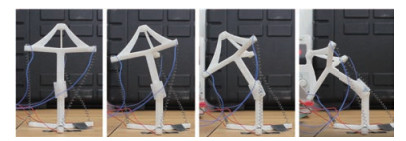图 6 GS-Bot机器人实验图 Fig.6 Experimental images of GS-Bot GS-Bot机器人可操控性验证实验总时长约30 s，通过高帧率图片可以发现，GS-Bot机器人的姿态改变主要集中在实验后10 s，形变趋势与图 4中SMA的形变特性相吻合。另外，将一个微型摄像机固定在机器人顶端用于观测外界环境并进行反馈，编写可视化模块对机器人进行实时状态反馈，并集成到目标检测软件中。 图 7所示，在软件中点击“测试1号SMA”按钮并点击“开始”，可观测到1号SMA驱动器开始弯曲变形。点击“目标检测”按钮，软件图形界面即显示实时的摄像机图像，“运动控制输入图像”显示实时目标检测结果和检测信息 (x, y, w, h)$$ x $$y$$ w  h $分别表示目标物左上角点在相机坐标系中的坐标值以及目标物的宽度和高度。GS-Bot机器人的可操控性实验验证了所设计的GS-Bot机器人具有可操控性和较好的运动形变性能。为进一步实现软体机器人智能规划控制打下基础。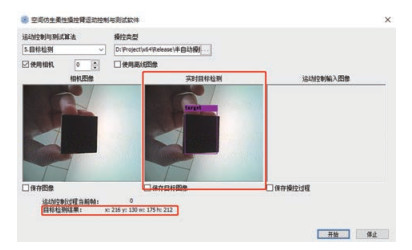图 7 GS-Bot软件可视化界面图 Fig.7 Visual interface of GS-Bot software 4.2 Q学习仿真结果 在仿真实验中，设定最大训练步数$ T^{'}_{\rm m} = $2000，最大训练周期数$ E_{\rm m} = $500，衰减因子$ \gamma = $0.9，神经网络隐藏单元数$ U= $1024。同时为了保证算法的泛化效果，取随机探索概率$ e= $0.95，即智能体在$ e $的概率下按照式(6) 输出对应动作，以$ 1-e $的概率选择随机动作$ a\in (-180^{{\circ}}, 180^{{\circ}}) $作为输出。仿真实验上位机CPU为Core i7-8700（8 GB），GPU为GTX-1060Ti（8 GB）。 仿真过程中机器臂运动轨迹如图 8所示，各子图分别表示机器臂在不同时刻的位置和姿态。其中图 8(a)图 8(b)表示机器臂末端不断试探触碰蓝色目标物体，图 8(c)表示经过一定时间的探索和学习，末端在到达并停留于目标物固定时长后，结束本回合训练。智能体通过式(10) 判断当前状态是否符合回合结束的设定条件。该仿真模型还可拓展至3节或多节机器人，如图 9所示。图 8 简化GS-Bot模型仿真运动示意图 Fig.8 Simulated motion diagram of the simplified GS-Bot model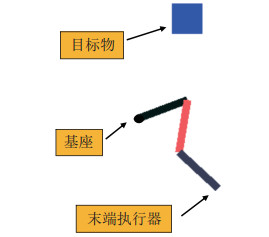图 9 三节模型仿真运动示意图 Fig.9 Simulated motion diagram of the three-section model 在实验结果中，为反映出智能体在仿真环境中的收敛趋势，本文给出了智能体在每个训练回合$ E_{\rm m} $中的累计奖励值和回合训练结束时训练步数的量化曲线，结果如图 10所示。可见智能体在训练100回合左右时，累计奖励值和训练步数已经趋于稳定。收敛后的智能体累计奖励值主要集中在30~50区域，训练稳定后训练步数主要集中在100~150步左右时。仿真结果验证了GS-Bot仿真模型在自主训练后能够在较短时间内迅速收敛到稳定状态，智能体在仿真环境中能够根据奖励函数的引导自主完成规划和控制任务。同时，为了验证隐藏单元数对智能体训练的影响，本文对隐藏层数量为128、1024和2048的情形分别进行了实验。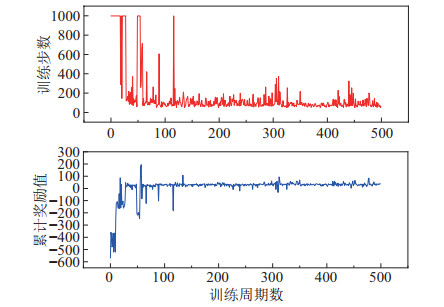图 10 仿真训练中奖励和训练步数曲线 Fig.10 Curves of reward and training steps in training simulation 分别随机选取5次训练结果，定义在最大训练步数$ T^{'}_{\rm m}= \$ 2000以内结束的回合为成功回合，单次训练回合成功率定义为成功回合数\!/\!总回合数500。由此统计不同隐藏层数量下训练的成功率，如表 1所示。表 1 不同隐藏层数的5次训练回合成功率 Tab. 1 Success rate after 5 rounds of training with different hidden layers

5 结论（Conclusion）

  Kim S, Laschi C, Trimmer B. Soft robotics: A bioinspired evolution in robotics[J]. Trends in Biotechnology, 2013, 31(5): 287-294. DOI:10.1016/j.tibtech.2013.03.002  Boyraz P, Runge G, Raatz A. An overview of novel actuators for soft robotics[J]. Actuators, 2018, 7(3). DOI:10.3390/act7030048  Pfeifer R, Lungarella M, Iida F. Self-organization, embodiment, and biologically inspired robotics[J]. Science, 2007, 318(5853): 1088-1093. DOI:10.1126/science.1145803  Jing Z L, Qiao L F, Pan H, et al. An overview of the configu-ration and manipulation of soft robotics for on-orbit servicing[J]. Science China: Information Sciences, 2017, 60. DOI:10.1007/s11432-016-9033-0  Laschi C, Cianchetti M, Mazzolai B, et al. Soft robot arm inspired by the octopus advanced robotics[J]. Advanced Robotics, 2012, 26(7): 709-727. DOI:10.1163/156855312X626343  Lin H T, Leisk G G, Trimmer B. GoQBot: A caterpillar-inspired soft-bodied rolling robot[J]. Bioinspiration & Biomimetics, 2011, 6(2). DOI:10.1088/1748-3182/6/2/026007  Shepherd R F, Ilievski F, Choi W, et al. Multigait soft robot[J]. Proceedings of the National Academy Sciences of the United States of America, 2011, 108(51): 20400-20403. DOI:10.1073/pnas.1116564108  Fang H B, Zhang Y T, Wang K W. Origami-based earthworm-like locomotion robots[J]. Bioinspiration & Biomimetics, 2017, 12(6). DOI:10.1088/1748-3190/aa8448  Kim B, Park S, Jee C Y, et al. An earthworm-like locomotive mechanism for capsule endoscopes[C]//IEEE/RSJ International Conference on Intelligent Robots and Systems. Piscataway, USA: IEEE, 2005. DOI: 10.1109/IROS.2005.1545608.  Seok S, Onal C D, Wood R, et al. Peristaltic locomotion with antagonistic actuators in soft robotics[C]//IEEE International Conference on Robotics and Automation. Piscataway, USA: IEEE, 2010. DOI: 10.1109/ROBOT.2010.5509542.  Ilievski F, Mazzeo A D, Shepherd R F, et al. Soft robotics for chemists[J]. Angewandte Chemie, 2011, 50(8): 1890-1895. DOI:10.1002/anie.201006464  Bar-Cohen Y. Electroactive polymer (EAP) actuators as artificial muscles: Reality, potential, and challenges[M]. 2nd ed. Bellingham, USA: SPIE, 2004.  Liu L W, Li J R, Lü X F, et al. Progress in constitutive theory and stability research of electroactive dielectric elastomers[J]. Scientia Sinica: Technologica, 2015, 45(5): 450-463.  Lampani L, Gaudenzi P. Finite element modeling for dielectric elastomer actuators (DEA)[C]//19th International Conference on Adaptive Structures and Technologies. Piscataway, USA: IEEE, 2008: 65-83.  Daerden F, Lefeber D. Pneumatic artificial muscles: Actuators for robotics and automation[J]. European Journal of Mechanical and Environmental Engineering, 2002, 1: 11-21.  Boblan I, Bannasch R, Schwenk H, et al. A human-like robot hand and arm with fluidic muscles: Biologically inspired construction and functionality[M]. Lecture Notes in Computer Science, Vol. 3139. Berlin, Germany: Springer, 2004: 160-179.  Mosadegh B, Polygerinos P, Keplinger C, et al. Pneumatic networks for soft robotics that actuate rapidly[J]. Advanced Functional Materials, 2014, 24(15): 2163-2170.  Li C Q, Rahn C D. Design of continuous backbone, cable-driven robots[J]. Journal of Mechanical Design, 2002, 124(2): 265-271.  Camarillo D B, Milne C F, Carlson C R, et al. Mechanics mode-ling of tendon-driven continuum manipulators[J]. IEEE Transactions on Robotics, 2008, 24(6): 1262-1273.  Follador M, Cianchetti M, Arienti A, et al. A general method for the design and fabrication of shape memory alloy active spring actuators[J]. Smart Materials and Structures, 2012, 21(11). DOI:10.1088/0964-1726/21/11/115029  Redmon J, Farhadi A. YOLOv3: An incremental improvement[DB/OL]. (2018-04-08)[2020-03-21]. https://arxiv.org/abs/1804.02767.